Cross Reference Down

Phatarfod, R (2013). Some aspects of the Benford law of leading significant digits. The Mathematical Scientist, Applied Probability Trust, 38 (2), pp 73-85.

This work cites the following items of the Benford Online Bibliography:

 Benford, F (1938). The law of anomalous numbers. Proceedings of the American Philosophical Society, Vol. 78, No. 4 (Mar. 31, 1938), pp. 551-572.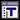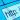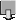Berger, A (2010). Large spread does not imply Benford's Law. Technical Report, Dept. of Mathematical and Statistical Sciences, University of Alberta, Edmonton, AB, Canada.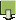Berger, A, Bunimovich, LA and Hill, TP (2005). One-dimensional dynamical systems and Benford's law. Transactions of the American Mathematical Society 357(1), pp. 197-219. ISSN/ISBN:0002-9947. DOI:10.1090/S0002-9947-04-03455-5.Berger, A and Hill, TP (2011). Benford's Law Strikes Back: No Simple Explanation in Sight for Mathematical Gem. The Mathematical Intelligencer 33(1), pp. 85-91. DOI:10.1007/ s00283-010-9182-3.Buck, B, Merchant, AC and Perez, SM (1993). An illustration of Benfords first digit law using alpha decay half lives. European Journal of Physics 14, pp. 59-63.Feller, W (1971). An Introduction to Probability Theory and Its Applications. 2nd ed., J. Wiley (see p 63, vol 2).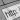Goudsmit, SA and Furry, WH (1944). Significant figures of numbers in statistical tables. Nature 154(3921), pp. 800-801. ISSN/ISBN:0028-0836. DOI:10.1038/154800a0.Hill, TP (1995). A Statistical Derivation of the Significant-Digit Law. Statistical Science 10(4), pp. 354-363. ISSN/ISBN:0883-4237.Hill, TP (1995). Base-Invariance Implies Benford's Law. Proceedings of the American Mathematical Society 123(3), pp. 887-895. ISSN/ISBN:0002-9939. DOI:10.2307/2160815.Hill, TP (1998). The First-Digit Phenomenon. American Scientist 86 (4), pp. 358-363. ISSN/ISBN:0003-0996. DOI:10.1511/1998.4.358.Matthews, R (1999). The Power of One. New Scientist 163, July 10, pp. 26-30.Newcomb, S (1881). Note on the frequency of use of the different digits in natural numbers. American Journal of Mathematics 4(1), pp. 39-40. ISSN/ISBN:0002-9327. DOI:10.2307/2369148.Pinkham, RS (1961). On the Distribution of First Significant Digits. Annals of Mathematical Statistics 32(4), pp. 1223-1230. ISSN/ISBN:0003-4851.Raimi, RA (1976). The First Digit Problem. American Mathematical Monthly 83(7), pp. 521-538. ISSN/ISBN:0002-9890. DOI:10.2307/2319349.Rodriguez, RJ (2004). First Significant Digit Patterns from Mixtures of Uniform Distributions. American Statistician 58(1), pp. 64-71. ISSN/ISBN:0003-1305. DOI:10.1198/0003130042782.Ross, KA (2011). Benford's Law, a growth industry. American Mathematical Monthly 118 (7), pp. 571-583. ISSN/ISBN:0002-9890. DOI:10.4169/amer.math.monthly.118.07.571.Stewart, I (1993). The half-life of a dirty book. New Scientist 138(1872), p. 12 . ISSN/ISBN:02624079.Stigler, GJ (1945). The distribution of leading digits in statistical tables. University of Chicago, Regenstein Library, Special Collections, George J. Stigler Archives.Weaver, W (1963). The distribution of first significant digits. pp 270-277 in: Lady Luck: The Theory of Probability, Doubleday Anchor Series, New York. Republished by Dover, 1982. ISSN/ISBN:978-0486243429.